March 23, 2021

## Outline

1. Example
2. Confounding
• definition
• sources of bias
• graphs
• when do we get confounding?

## Example:

Karaivanov et al (2020), economists at SFU, investigate:

Have indoor mask mandates reduced COVID cases, on average?

## Example:

They compare COVID cases in Ontario Public Health Units (PHU) with and without mask mandates

• Correlation of mask mandate and COVID cases

## Example: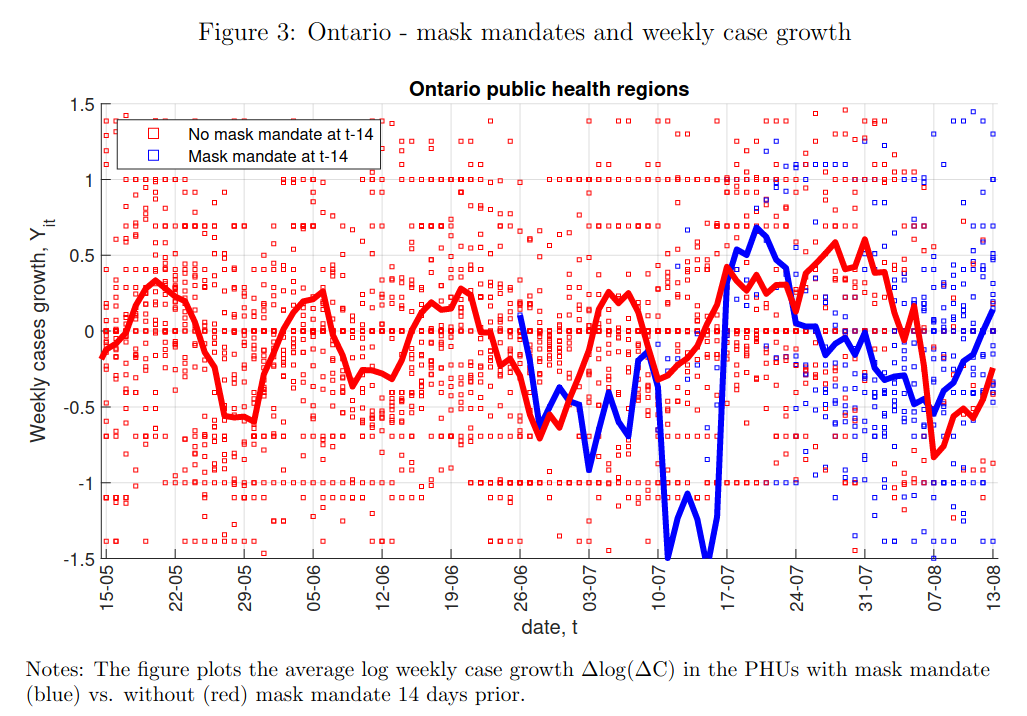poll

## Example:Why doesn’t correlation imply causation?

## Confounding

Correlation suffer from two sources of error:

random error: we observe patterns in $$X$$ (independent variable) and $$Y$$ (dependent variable) by chance, when there is in fact no relationship.

bias (confounding): the observed pattern between $$X$$ and $$Y$$ is not the true causal relationship between $$X$$ and $$Y$$.

## Confounding

• What is confounding?
• Why does it happen?
• What circumstances make it happen?
• What is the direction of the bias it produces?

## Confounding

confounding is when there is a systematic observed correlation between $$X$$ and $$Y$$ that is does NOT reflect the causal effect of $$X$$ on $$Y$$.

• This is not a chance correlation.
• Two ways to explain why this happens (different explanations, but two sides of the same coin)

## Confounding

### Explanation 1:

Confounding happens when the cases we observe with different levels of $$X$$ have different (factual and counterfactual) potential outcomes of $$Y$$.

Example: Imagine we want to know whether mask mandate causes PHU to have lower COVID cases. Because of FPCI, we use correlation:

• compare PHU 1 (with mask mandate) to PHU 2 (without)

## Confounding

In correlation, assume that PHU 1 without mask mandate (counterfactual) is the same as PHU 2 without mask mandate (factual)

PHU Mask Mandate No Mandate
1 $$\mathrm{COVID \ Cases_{PHU \ 1}(Mandate)}$$ $$\color{red}{\mathrm{COVID \ Cases_{PHU \ 1}(No \ Mandate)}}$$
$$\Downarrow{=}$$ $$\Uparrow{=}$$
2 $$\color{red}{\mathrm{COVID \ Cases_{PHU \ 2}(Mandate)}}$$ $$\mathrm{COVID \ Cases_{PHU \ 2}(No \ Mandate)}$$

## Confounding

If this substitution is wrong: PHU 1 and 2 have different factual/counterfactual COVID caseloads, correlation is biased.

PHU Mask Mandate No Mandate
1 $$\boxed{\mathrm{COVID \ Cases_{PHU \ 1}(Mandate)}}$$ $$\color{red}{\mathrm{COVID \ Cases_{PHU \ 1}(No \ Mandate)}}$$
$$\Downarrow{\neq}$$ $$\Uparrow{\neq}$$
2 $$\color{red}{\mathrm{COVID \ Cases_{PHU \ 2}(Mandate)}}$$ $$\boxed{\mathrm{COVID \ Cases_{PHU \ 2}(No \ Mandate)}}$$

## Confounding

Why do these two PHUs have different potential outcomes?

• There other differences besides mask mandate…

### Explanation 2:

Confounding occurs when these other differences between cases (third variables, e.g. $$W$$) causally affect $$X$$ and $$Y$$.

This can be understood visually

## Causal Graphs

Causal graphs represent a model of the true causal relationships between variables.

the nodes or dots correspond to variables

• can be labeled with generic names for independent/dependent variables ($$X$$, $$Y$$) or meaningful names (e.g. “Mask Mandate”, “COVID Cases”)

the arrows convey the direction of causality

• $$X \rightarrow Y$$ means that $$X$$ causes changes in $$Y$$
• $$X \leftarrow W$$ means that $$W$$ causes changes in $$X$$

## Causal Graphs

### For example

PHU 1 (that had a mask mandate) may have a larger population of university educated adults than PHU 2 (no mask mandate).

• More educated residents might be more likely to work from home $$\xrightarrow{}$$ mask mandate affects fewer people $$\xrightarrow{}$$ more likely to implement.
• More educated residents $$\xrightarrow{}$$ work from home $$\xrightarrow{}$$ fewer contacts $$\xrightarrow{}$$ lower COVID cases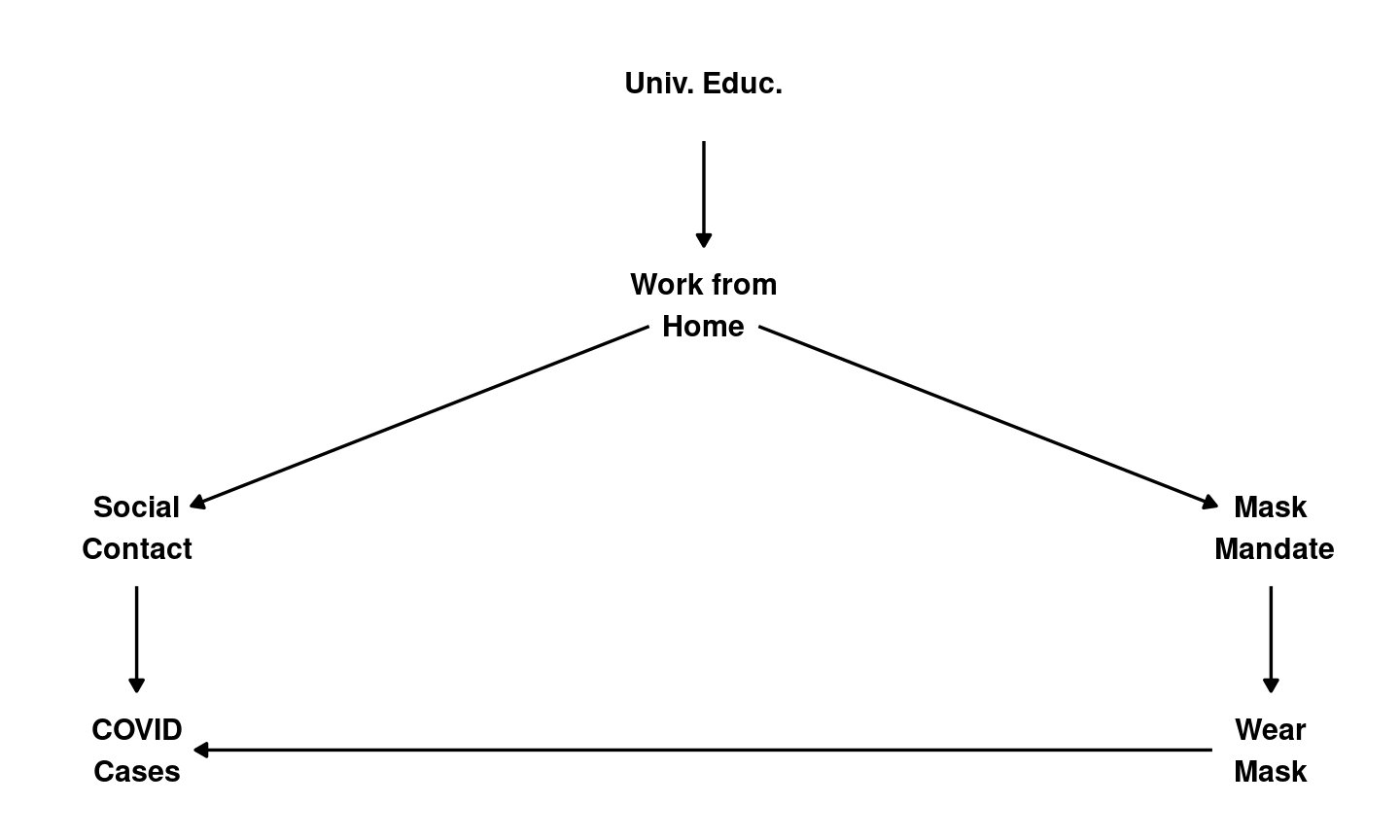## Causal Graphs

In a causal graph, there is confounding of correlation of $$X$$ and $$Y$$ if…

1. some variable $$W$$ has causal paths toward $$X$$ and $$Y$$
2. (equivalently) there is backdoor path or non-causal path from $$X$$ to $$Y$$
• a chain of two or more arrows that follows arrows backwards out of $$X$$, changes direction once and follows arrows toward $$Y$$: $$X \leftarrow W \leftarrow Z \rightarrow Y$$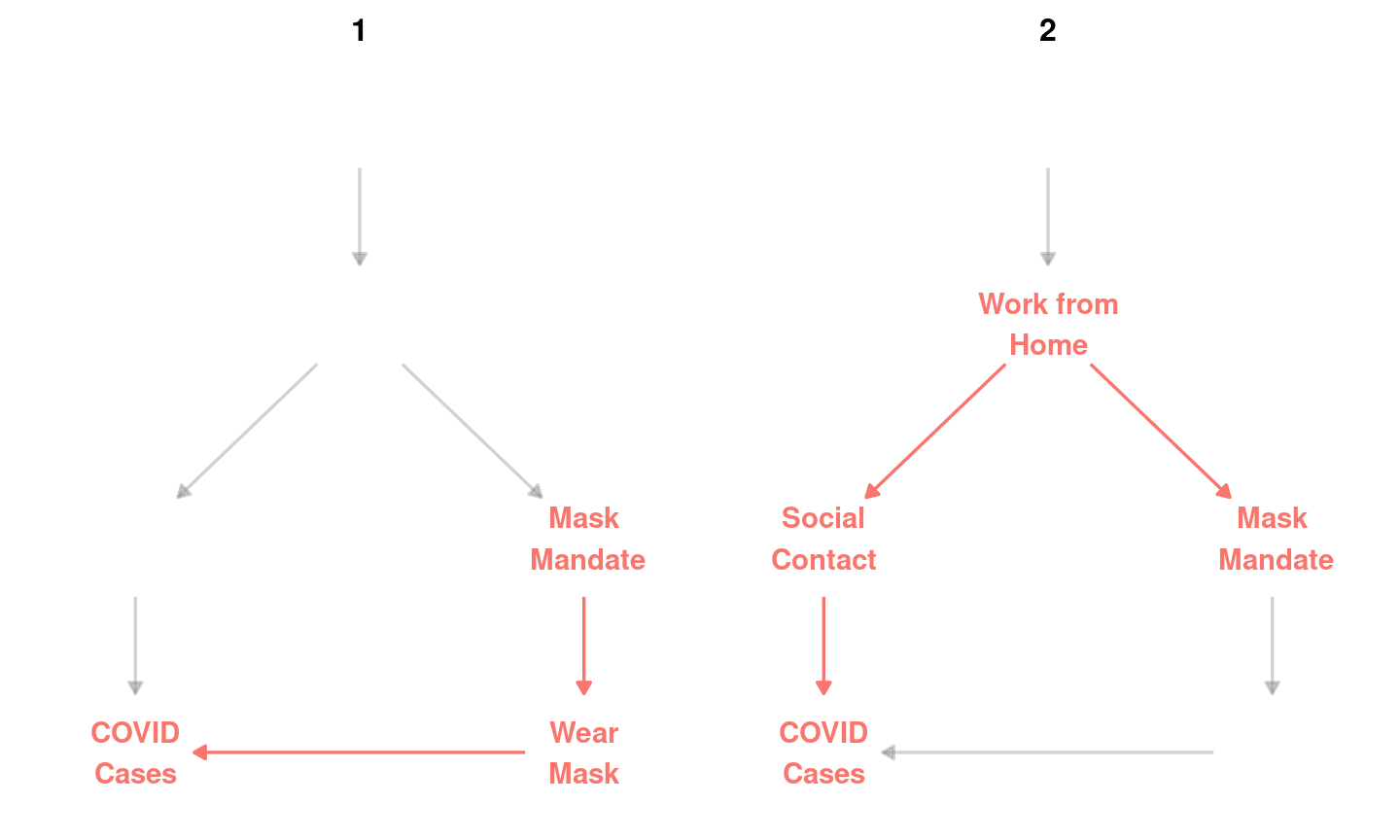## Causal Graphs: Confounding

In reality, we don’t really know the variables and paths on these causal graphs.

Instead, these causal graphs help us think about possible scenarios that might produce bias/confounding of the correlation between $$X$$ and $$Y$$.

## Activity

In groups…

Imagine you look at the correlation between mask mandates and COVID Cases…

given the correlation you observe, propose a causal graph that would imply that the correlation is the result of confounding.

## Confounding:

These examples illustrate the possibility that if causal graphs include variables in addition to the independent and dependent variables, there is a risk of confounding or bias.

Do all additional variables produce confounding?

No… We will discuss three different patterns of variables: some of which have confounding, some which do not.

## Additional Variables: Patterns

• antecedent variables
• sometimes confounding
• sometimes no confounding
• intervening variables
• no confounding
• reverse causality
• yes, confounding.

## Antecedent Variables

antecedent variable: a variable that affects $$X$$

• e.g. in this path, $$W \xrightarrow{} X \xrightarrow{} Y$$, $$W$$ is an antecedent variable.

• antecedent variables ($$W$$) do not produce confounding if the only causal path from $$W$$ to $$Y$$ passes through $$X$$.
• antecedent variables do produce confounding if there is another causal path from $$W$$ to $$Y$$ that does NOT include $$X$$.

## Antecedent Variable: Confounding?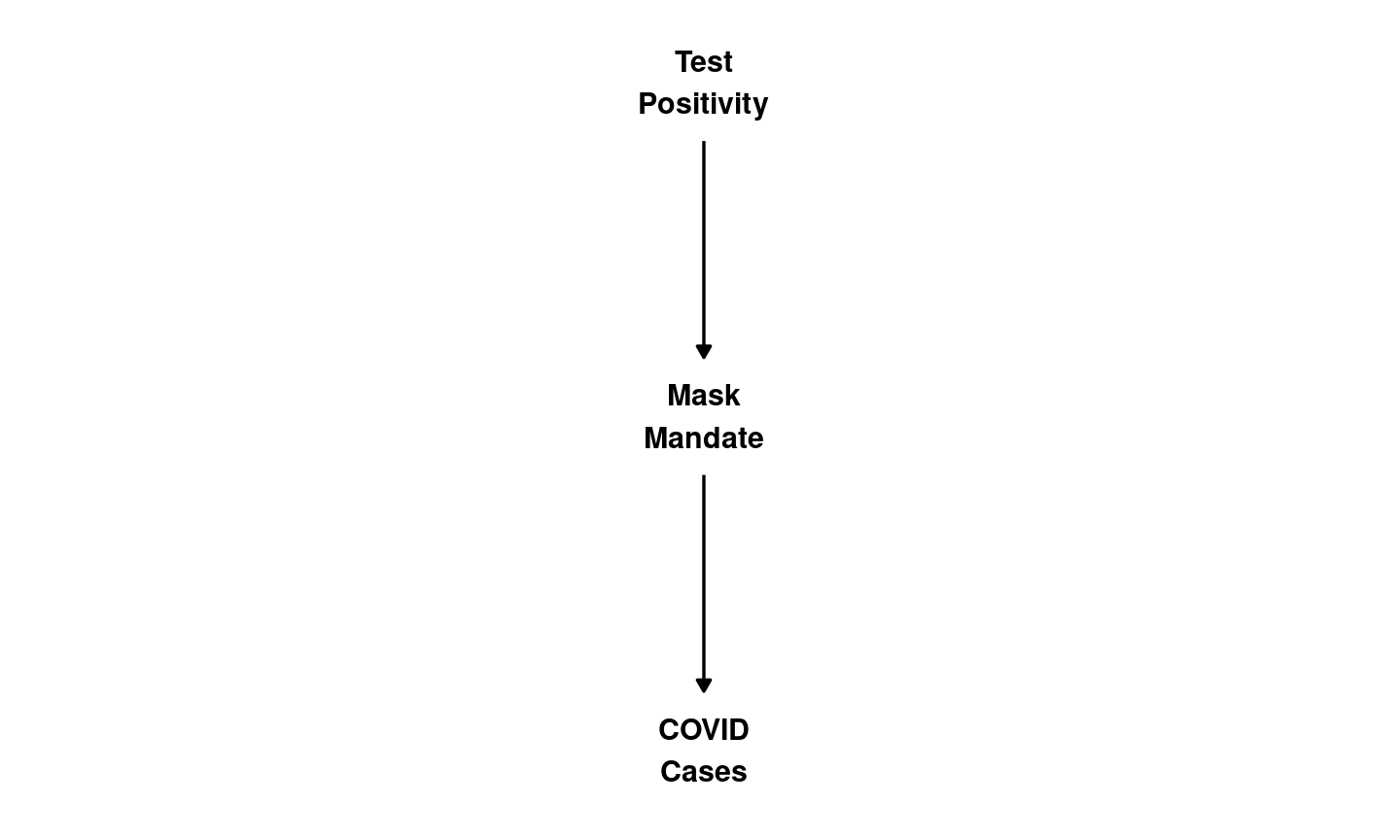• No. No “backdoor” path.

## Antecedent Variable: Confounding?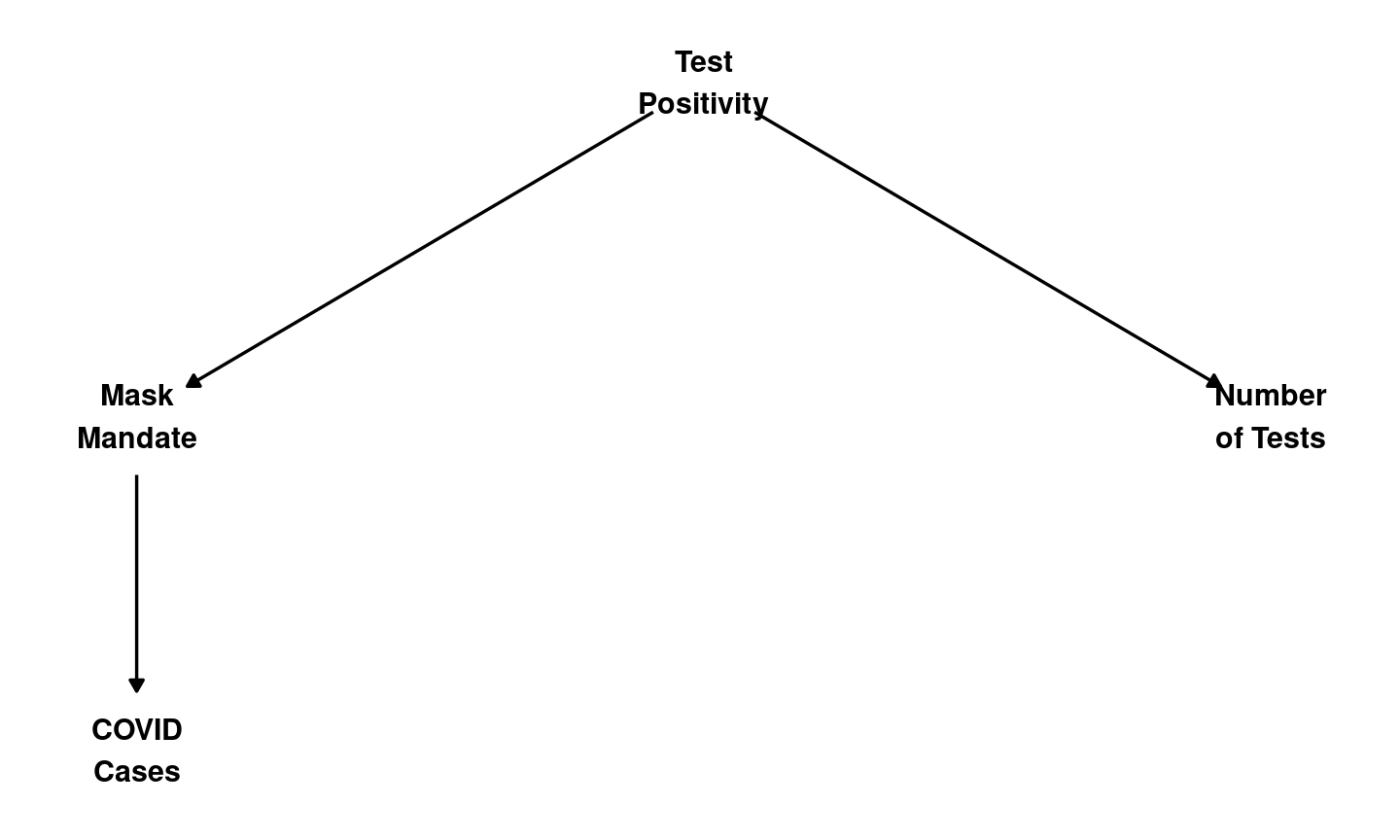• No. No “backdoor” path.

## Antecedent Variable: Confounding?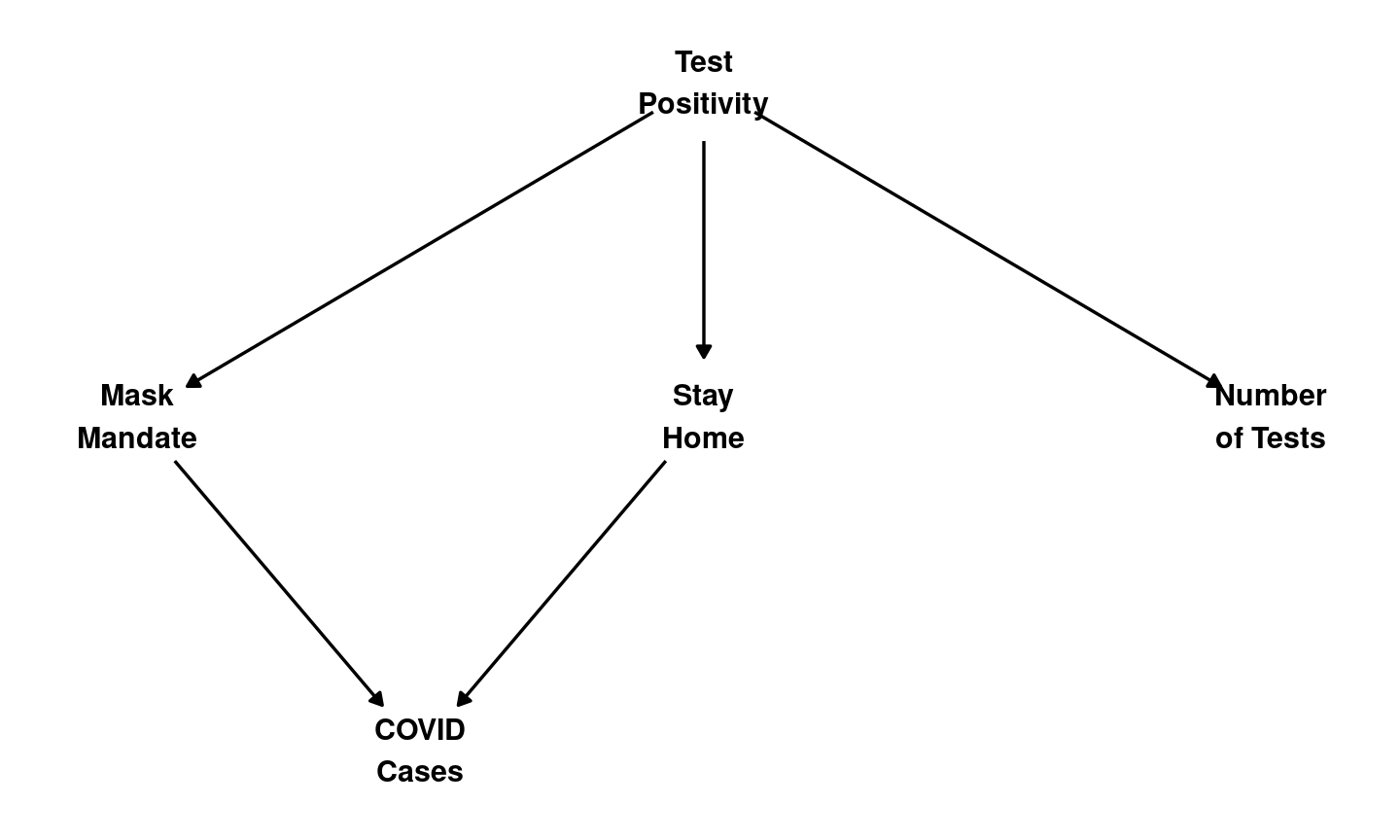• Yes. Mandate $$\xleftarrow{}$$ Positives $$\xrightarrow{}$$Stay Home$$\xrightarrow{}$$COVID Cases

## Antecedent Variable: Confounding?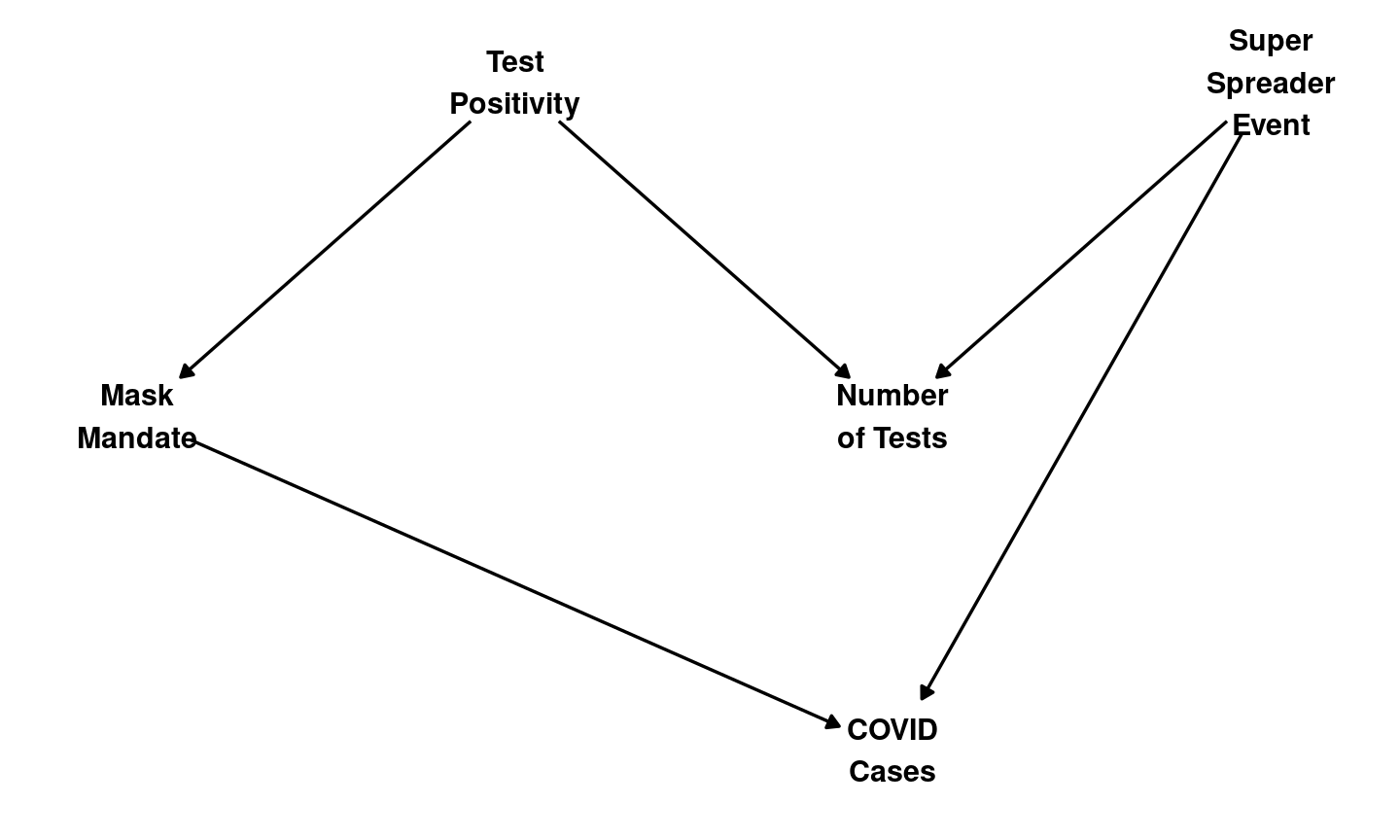• No. No “backdoor” path; apparent “backdoor” changes directions more than once.

## Intervening Variables

intervening variable: a variable that affects $$Y$$ and is affected by $$X$$.

• e.g. in this path, $$X \xrightarrow{} M \xrightarrow{} Y$$, $$M$$ is an intervening variable.

• intervening variables ($$M$$) do not produce confounding because they are on the causal path from $$X$$ to $$Y$$. They do not produce backdoor path.

## Intervening Variable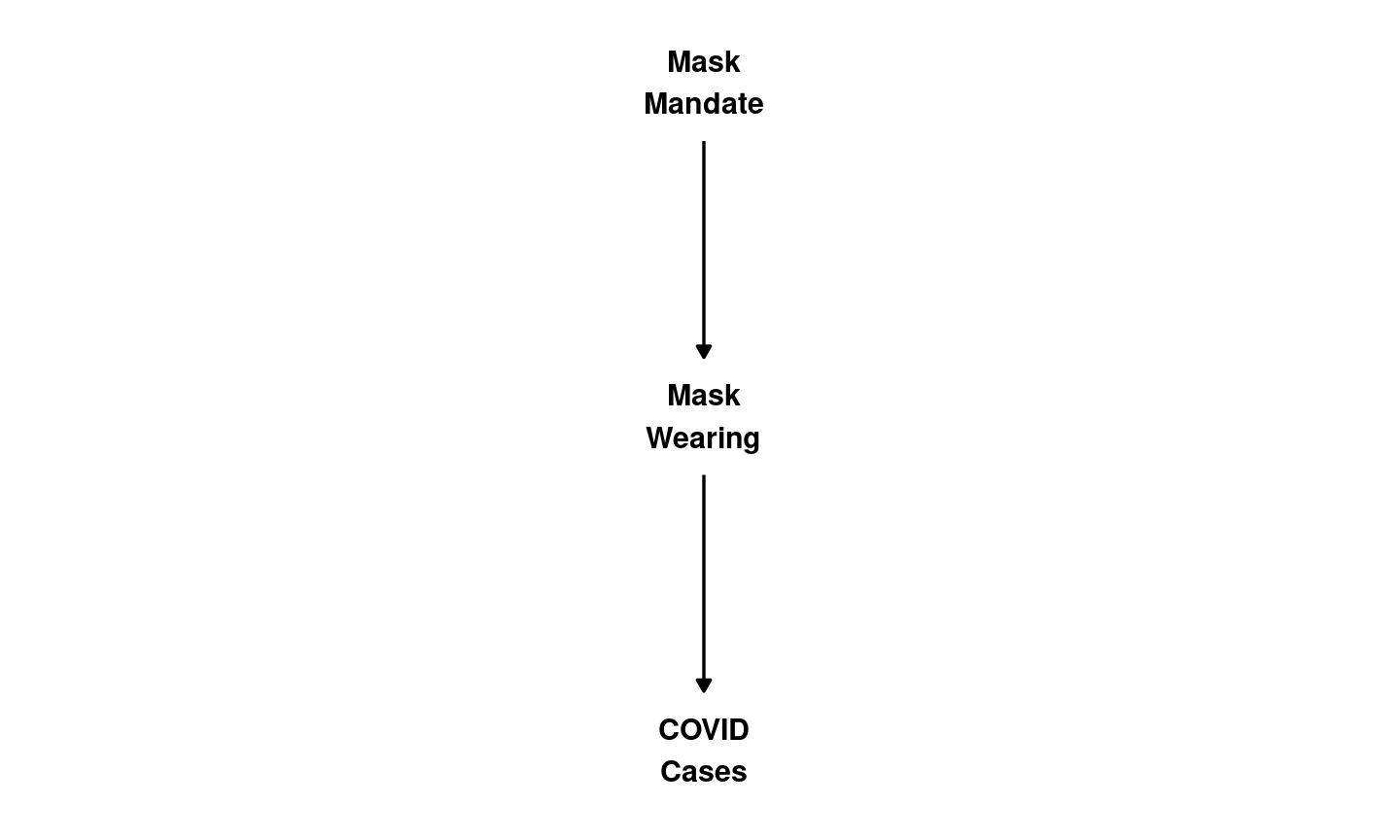• No backdoor path. Mask mandate affects COVID through mask wearing.

## Reverse Causality

reverse causality describes the situation where the dependent variable $$Y$$ actually causes the independent variable $$X$$.

So while we use the correlation to describe the effect of $$X$$ on $$Y$$: $$X \to Y$$, the correlation in fact is the result of the effect of $$Y$$ on $$X$$: $$Y \to X$$.

This is a special case of bias or confounding.

Third Variable? Key Attribute Confounding?
Antecedent Variables
($$W$$)
Yes $$W \to X$$ If only causal path from $$W$$ to $$Y$$ contains $$X$$: No
If a causal path from $$W$$ to $$Y$$ excludes $$X$$: Yes
Intervening Variables ($$M$$) Yes $$X \to M \to Y$$ No
Reverse Causality No $$Y \to X$$ Yes

## Conclusion

Confounding

1. bias: observed correlation $$\neq$$ true casual relationship
2. Why?
• cases with different values of $$X$$, different in other ways
• if “third” variable affects $$X$$, affects $$Y$$ $$\to$$ confounding.
3. Causal graphs help us diagnose possible sources of confounding.

Next: Direction/Size of bias; turning correlation into causation.